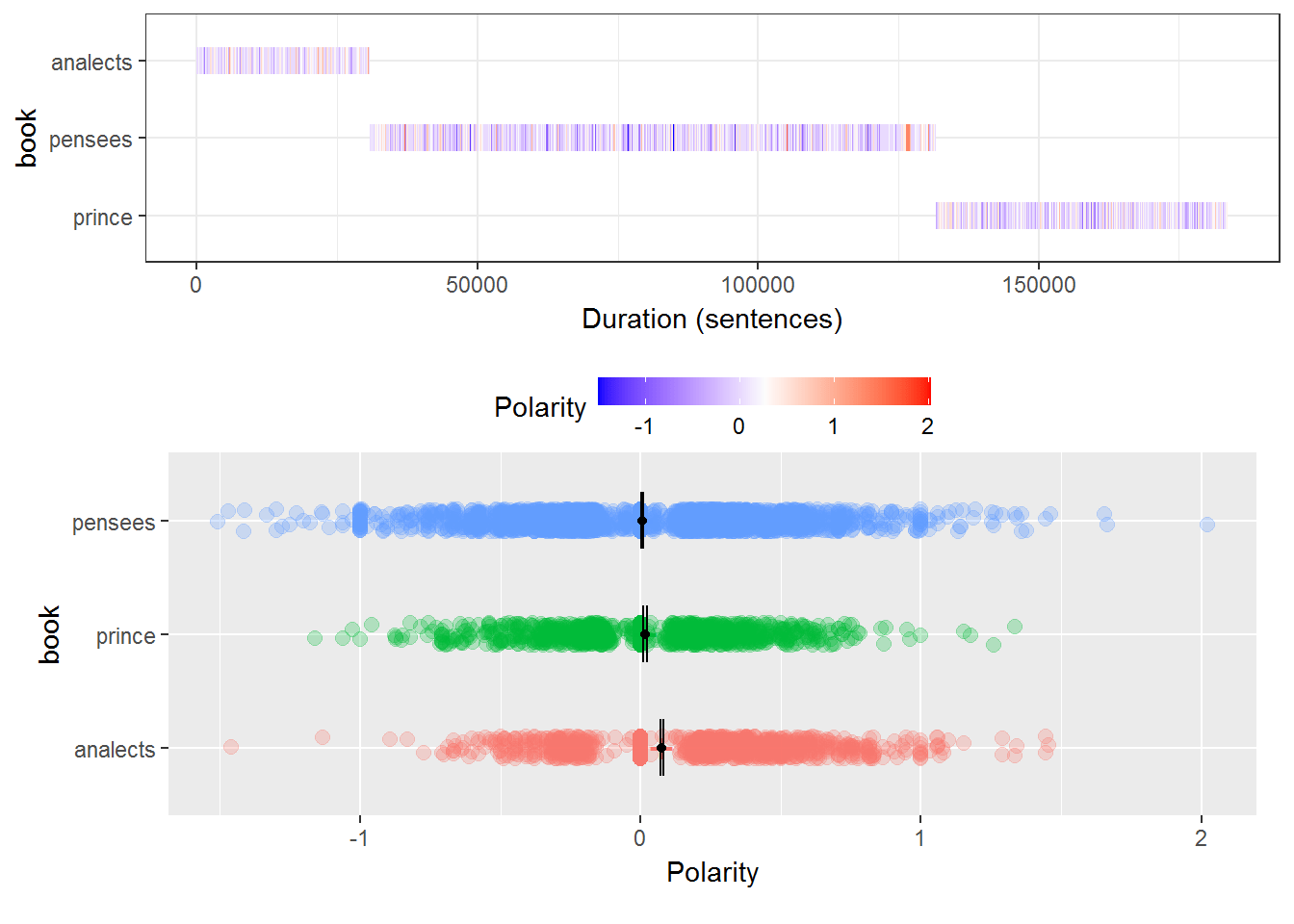# Sentiment Analysis in R

In this post, we will perform a sentiment analysis in R. Sentiment analysis involves employs the use of dictionaries to give each word in a sentence ascore. A more positive word is given a higher positive number while a more negative word is given a more negative number. The score is then calculated based on the position of the word, the weight, as well as other more complex factors. This is then performed for the entire corpus to give it a score.

We will do a sentiment analysis in which we will compare three famous philosophical texts

• Analects
• The Prince
• Penesees

These books are available at the Gutenberg Project. You can go to the site type in the titles and download them to your computer.

We will use the “qdap” package in order to complete the sentiment analysis. Below is some initial code.

``library(qdap)``

Data Preparation

Below are the steps we need to take to prepare the data

1. Paste the text files into R
2. Convert the text files to ASCII format
3. Convert the ASCII format to data frames
4. Split the sentences in the data frame
5. Add a variable that indicates the book name
6. Combine the three books into one dataframe

We now need to prepare the three text. First, we move them into R using the “paste” function.

``````analects<-paste(scan(file ="C:/Users/darrin/Documents/R/R working directory/blog/blog/Text/Analects.txt",what='character'),collapse=" ")
pensees<-paste(scan(file ="C:/Users/darrin/Documents/R/R working directory/blog/blog/Text/Pascal.txt",what='character'),collapse=" ")
prince<-paste(scan(file ="C:/Users/darrin/Documents/R/R working directory/blog/blog/Text/Prince.txt",what='character'),collapse=" ")``````

We need to convert the text files to ASCII format see that R is able to read them.

``````analects<-iconv(analects,"latin1","ASCII","")
pensees<-iconv(pensees,"latin1","ASCII","")
prince<-iconv(prince,"latin1","ASCII","")``````

Now we make our dataframe for each book. The argument “texts” gives our dataframe one variable called “texts” which contains all the words in each book. Below is the code data frame

``````analects<-data.frame(texts=analects)
pensees<-data.frame(texts=pensees)
prince<-data.frame(texts=prince)``````

With the dataframes completed. We can now split the variable “texts” in each dataframe by sentence. We will use the “sentSplit” function to do this.

``analects<-sentSplit(analects,'texts')``
``pensees<-sentSplit(pensees,'texts')``
``prince<-sentSplit(prince,'texts')``

Next, we add the variable “book” to each dataframe. What this does is that for each row or sentence in the dataframe the “book” variable will tell you which book the sentence came from. This will be valuable for comparative purposes.

``````analects\$book<-"analects"
pensees\$book<-"pensees"
prince\$book<-"prince"``````

Now we combine all three books into one dataframe. The data preparation is now complete.

``threebooks<-rbind(analects,pensees,prince)``

Data Analysis

We are now ready to perform the actual sentiment analysis. We will use the “polarity” function for this. Inside the function, we need to use the text and the book variables. Below is the code. polarity analysis

``pol<-polarity(threebooks\$texts,threebooks\$book)``

We can see the results and a plot in the code below.

``pol``
``````##       book total.sentences total.words ave.polarity sd.polarity stan.mean.polarity
## 1 analects            3425       31383        0.076       0.254              0.299
## 2  pensees            7617      101043        0.008       0.278              0.028
## 3   prince            1542       52281        0.017       0.296              0.056``````

The table is mostly self-explanatory. We have the total number of sentences and words in the first two columns. Next is the average polarity and the standard deviation. Lastly, we have the standardized mean. The last column is commonly used for comparison purposes. As such, it appears that Analects is the most positive book by a large margin with Pensees and Prince be about the same and generally neutral.

``plot(pol)``The top plot shows the polarity of each sentence over time or through the book. The bluer the more negative and the redder the more positive the sentence. The second plot shows the dispersion of the polarity.

There are many things to interpret from the second plot. For example, Pensees is more dispersed than the other two books in terms of polarity. The Prince is much less dispersed in comparison to the other books.

Another interesting task is to find the most negative and positive sentence. We need to take information from the “pol” dataframe and then use the “which.min” function to find the lowest scoring. The “which.min” function only gives the row. Therefore, we need to take this information and use it to find the actual sentence and the book. Below is the code.

``````pol.df<-pol\$all #take polarity scores from pol.df
which.min(pol.df\$polarity) #find the lowest scored sentence``````
``##  6343``
``pol.df\$text.var #find the actual sentence``
``##  "Apart from Him there is but vice, misery, darkness, death, despair."``
``pol.df\$book #find the actual book name``
``##  "pensees"``

Pensees had the most negative sentence. You can see for yourself the clearly negative words which are vice, misery, darkness, death, and despair. We can repeat this for the most positive sentence

``which.max(pol.df\$polarity)``
``##  4839``
``pol.df\$text.var``
``##  "You will be faithful, honest, humble, grateful, generous, a sincere friend, truthful."``
``pol.df\$book``
``##  "pensees"``

Again Pensees has the most positive sentence with such words as faithful, honest, humble, grateful, generous, sincere, friend, truthful all being positive.

Conclusion

Sentiment analysis allows for the efficient analysis of a large body of text in a highly qualitative manner. There are weaknesses to this approach such as the dictionary used to classify the words can affect the results. In addition, Sentiment analysis only looks at individual sentences and not larger contextual circumstances such as a paragraph. As such, a sentiment analysis provides descriptive insights and not generalizations.